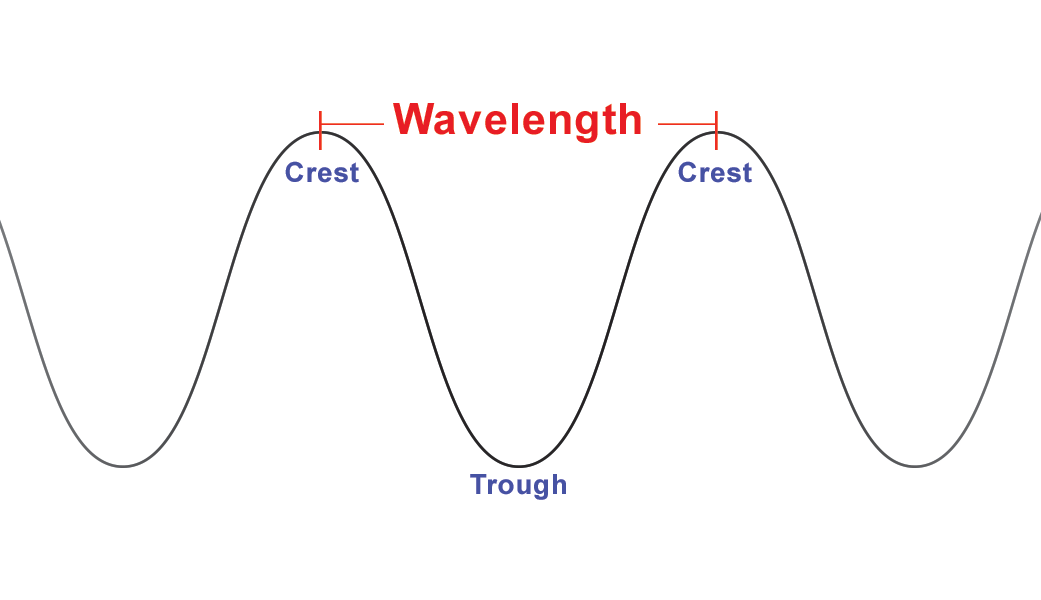الرئيسية » Simplified Chemistry » Nature of light and electromagnetic spectrum

# Nature of light and electromagnetic spectrum

Nature of light and electromagnetic spectrum:  According to Newton’s corpuscular theory, light is propagated as a stream of particles, called corpuscles. This could justify the phenomenon of reflection and refraction of light but failed to explain the phenomenon of diffraction, interference etc. Clerk Maxwell suggested that light waves are associated with both electric and magnetic fields, i.e. light may be described as  electromagnetic waves or radiations. there are three characteristics properties associated with any kind of wave:

1 –  Wavelength is the distance between the two neighboring crests or troughs of a wave. It is generally symbolized by the Greek letter lambda (λ).

2 – Frequency is the number of waves per second. It is denoted by the Greek letter nu (υ). It’s unit is cycle per second or Hertz (Hz).

3 –  Velocity is the distance travelled by the wave in one second. It is denoted by the letter c and is equal to the product of wavelength and frequency i.e.

c = λυ      or     υ = c/λ

the velocity of light 3×108 meters/second.

Dual nature of light: Light is propagated as ‘a stream of particles, called corpuscles which justifies its particle nature. Reflection and refraction of light justify this nature. But the phenomenon such as the interference, diffraction etc. lead to the fact that light possesses wave nature. This suggests that light behaves both like a particle and wave nature.مقالات قد تفيدك :

### عن Akram Amir El Aliاستاذ الكيمياء التحليلية ومصمم غرافيك